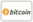Bookcover of Universal method for solving problems of linear algebra
Booktitle:

# Universal method for solving problems of linear algebra

## Method of elementary transformations

LAP LAMBERT Academic Publishing (2013-05-06 )eligible for voucher
ISBN-13:

### 978-3-659-38200-0

ISBN-10:
3659382000
EAN:
9783659382000
Book language:
English
Blurb/Shorttext:
The book presents the universal method for solving problems of linear algebra - the method of elementary transformations of matrices. It is shown in numerous examples how to solve the basic problems of linear algebra: solving systems of linear equations, calculation of the inverse matrix, linear dependence and span of vector systems, finding the eigenvalues and eigenvectors of a linear operator, kernel and image of a linear operator, bringing a polynomial matrix to a canonical form, finding the Jordan and the Frobenius forms of the matrix, reduction of a quadratic form to a sum of squares, various types of decomposition of matrices, finding an orthogonal basis of the subspace spanned by the given vector system, etc. The book is intended for students, studying the university course of linear algebra, as well as for teachers, conducting practical classes on the subject.
Publishing house:
Website:
https://www.lap-publishing.com/
By (author) :
Sergey Tyurin
Number of pages:
88
Published on:
2013-05-06
Stock:
Available
Category:
Mathematics
Price:
54.90 €
Keywords:
Linear algebra### Categories